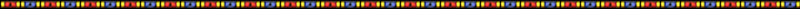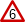The Wiki for Tale 6 is in read-only mode and is available for archival and reference purposes only. Please visit the current Tale 10 Wiki in the meantime.

If you have any issues with this Wiki, please post in #wiki-editing on Discord or contact Brad in-game.

# Gearbox Design GuideTale 4 information
• Do not assume any information on this page is valid for Tale 5
• If you can confirm information is valid for Tale 5, place the symbolnext to that item with the {{5ok}} tag## Overview

Gearboxes are used in a number of the more advanced buildings, normally to add a unique cost for each construction. Each gearbox takes an input shaft rotating and converts it via a gear train into up to 3 outputs. The process of designing a gearbox is to figure out which ratios are needed and to implement those ratios in the form of positioning various sizes of connected gears on a Gearbox Assembly Table.

## Gearbox design

### Gears

There are 5 gear sizes available, measured by their number of teeth (or relative number of teeth):

Gear Size Item Metal cost Wax cost CC cost (student) CC cost (master)
1 Spacer 0 0 0 0
3 Small Gear 2 Brass 2 1 0.8 (batch of 10)
4 Small Gear 2 Brass 2 1 0.8 (batch of 10)
5 Medium Gear 15 Iron 15 5 10
6 Medium Gear 15 Iron 15 5 10
7 Large Gear 100 Iron 100 N/A 40

### Constraints

Up to 15 shafts may be placed in the 8x8 grid on the gearbox assembly table. Each shaft may contain up to 3 gears or spacers, and a gearbox may have no more than 30 gears in total (including spacers). There must be at least one gear on the input shaft at 1A, and a connected set of gears leading to between 1 and 3 shafts on the output row (8A-8H, at the top). The output row 8 may contain a maximum of three occupied cells, presumably to be used as outputs - no extra transfer gear locations.

### Meshing

If two shafts are near each other, a gear on one shaft may mesh with a gear on the nearby shaft. This depends upon the sizes of the gears involved, and how far apart the shafts are.

• Adjacent: gear sizes must sum to 6 (the only possible combination is 3 meshing with 3)
• Diagonal: gear sizes must sum to 8 (4 meshes with 4, or 5 with 3)
• 2 steps apart: gear sizes must sum to 11 (6 meshes with 5, or 7 with 4)
• "Knight's move", 2 steps away then 1 sideways: sizes must sum to 12 (6 with 6, or 7 with 5)

To mesh, gears must also be on the same layer, i.e. both being the first gears on their respective shafts, both second, or both third. If the sum of sizes of gears between a pair of shafts is greater than needed to mesh, they are too close and you must remove one. This includes spacers, which count as greater than 1 (but less than 2) for meshing purposes. For example you can not put a spacer adjacent to a 5 or 6 gear, and you can not put a spacer either adjacent or diagonal to a 7 gear.

To minimize conflicts with adjacent shafts, generally put your small gears on the bottom layer with your medium and large gears on the second or third layer.

### Standard Gearbox Notation

• / meshes with.
```   example: 5/3 or (5/3) or 53 - a size 5 gear that meshes with a size 3 gear.
```
• * shares a shaft
```   example: (5/3) * (7/4) - a size 5 gear meshing with a size 3 gear. On the same shaft
as the size 3 gear is a size 7 gear, which meshes with a size 4 gear.
```
```   example: (7/4) * (5/3) - the 7 still meshes with the 4, and the 5 with the three,
but this time, the 4 and the 5 share a shaft. Note that this results in the same
output as the previous example - as long as all the same gears mesh, you can move
it around in the chain without affecting the output speed.
```

This notation gives a standardised way of representing the gearbox table. To get from the factored notation to the actual rotation rate, just multiply out the fractions. For example, the (5/3) * (7/4) combination above would give (5 * 7) / (3 * 4) = 35/12 = 2.91666666 (which, as a percentage, rounds to 292%)

When designing a gearbox it's often useful to use factored notation, (6/5 * 5/3) * 5/3 = 655/533 (meaning 6x5x5 divided by 5x3x3) rather than 333%, as it's much easier to visualize the common elements when using the factored notation.

### Rate in Gear Trains

Each shaft has a rotation rate, based on the 1A input shaft being 100%. In a properly designed gearbox, you can trace any output back to the input via a single chain of meshed gears between shafts. The rotation of the output is driven by the rotation of the second-last shaft, and so on back to 1A.

If the meshed gears between a pair of shafts are the same size, then both shafts rotate at the same rate. If they are not the same size, the shaft with the larger gear rotates slower. For example, suppose shaft 1A has a 3-gear (with 3 teeth), meshing with a 5-gear on shaft 2B. Every 5 rotations of shaft 1A will pass 15 teeth through where the gears mesh. Those 15 teeth are only 3 rotations of a 5-gear, so shaft 2B will rotate 3 times for every 5 rotations of 1A. That is, a rotation rate of (3/5) or 60%.

The same principle can be used many times in a row by using the 3 different layers available. A 3-gear on 1A can mesh with a 5-gear on 2B on the bottom layer, meaning that 2B rotates at 60%. Then we can put a 3-gear on the second layer of 2B, meshing with a 5-gear on the second layer of 3C (with a 1-spacer on the bottom layer). So 3C rotates at (3/5) of 2B, which is in turn (3/5) of shaft 1A. The final rotation rate of 3C is thus (3/5)*(3/5) = 36%.

### Putting it Together

The need for gears to mesh, together with how they determine rotation, means that any output can be expressed as a product of a limited set of fractions. The earlier example is (3/5)*(3/5), expressing that going from 1A to 2B we mesh a 3-gear with a 5-gear (in that order), and the same from 2B to 3C. To build a gearbox with outputs in a desired range, you just need to find a sequence of fractions that multiply to give the right numbers. Much of the work has been done already, with a list of common Gearbox Ratios that may be useful.

3/3 and 4/4 just carry a given rotation rate from shaft to shaft, and are comparatively cheap since gears of size 3 and 4 are small gears. Many designed gearboxes contain chains of gears -3-3-3 or -4-4-4 carrying a given ratio to the required output shaft.

3/5 and 5/3 are the next cheapest, since size 5 is a medium gear.

5/6 and 6/5 come next in cost order, with both 5 and 6 being medium gears.

3/6 and 6/3 are shorthand, with an implicit 5-gear in the middle since 3-gears and 6-gears cannot mesh directly. Hence this ratio costs 2 mediums and a small.

4/7 and 7/4 are much more expensive: a 7-gear is a Large gear costing 120 iron and requiring the master casting box.

More expensive still are the shorthand ratios of 3/7, 7/3, 6/7 and 7/6, all of which have an implicit 5-gear in the middle to allow the others to mesh. Finally there is 6/4 which has an implicit 5 and 7 in the middle. Fortunately these ratios are almost never needed.

The relative cost of the various gear ratios ignores the small gears and assumes that a large gear costs 5 times as much as a medium gear. A combination like (3/5*5/6) that has a shared gear will be a bit cheaper. Note that every change in speed requires a medium or large gear.

• Cost of 1 - 3/5 and 5/3
• Cost of 2 - 5/6 and 6/5
• Cost of 6 - 4/7 and 7/4 (or 5/7 and 7/5)